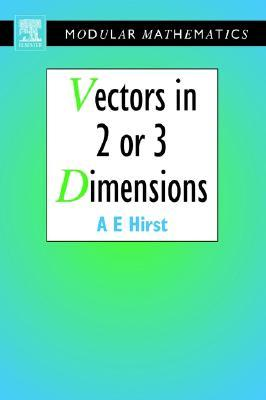Home » Vectors in Two or Three Dimensions by Ann Hirst# Vectors in Two or Three Dimensions

## Ann Hirst

Published August 31st 1995
ISBN : 9780340614693
Paperback
144 pages
Book Rating:Enter the sum

 About the Book Vectors in 2 or 3 Dimensions provides an introduction to vectors from their very basics. The author has approached the subject from a geometrical standpoint and although applications to mechanics will be pointed out and techniques from linear algebraMoreVectors in 2 or 3 Dimensions provides an introduction to vectors from their very basics. The author has approached the subject from a geometrical standpoint and although applications to mechanics will be pointed out and techniques from linear algebra employed, it is the geometric view which is emphasised throughout.Properties of vectors are initially introduced before moving on to vector algebra and transformation geometry. Vector calculus as a means of studying curves and surfaces in 3 dimensions and the concept of isometry are introduced later, providing a stepping stone to more advanced theories.* Adopts a geometric approach* Develops gradually, building from basics to the concept of isometry and vector calculus* Assumes virtually no prior knowledge* Numerous worked examples, exercises and challenge questions